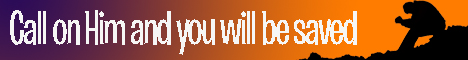Note:  Do not rely on this information. It is very old.

# Momentum

Momentum,. or, as it was called by Newton, the "quantity of motion" of a moving body, is the product of the mass of the body and its velocity. A force acting on a body for a certain time produces in that body a definite amount of momentum; and experiment has shown that the momentum is equal to the product of the force and the time during which it acts. When any body acts on a second, the latter reacts on the former, and the action and reaction are equal and opposite, as stated in Newton's third law of motion. The momentum produced by the action must therefore be equal to that produced by the reaction; this is equivalent to saying that there is no loss of momentum when two bodies act on each other. Applying this to any system of bodies mutually acting on each other, we see the momentum of the system remains constant. This fact is known as the conservation of momentum.